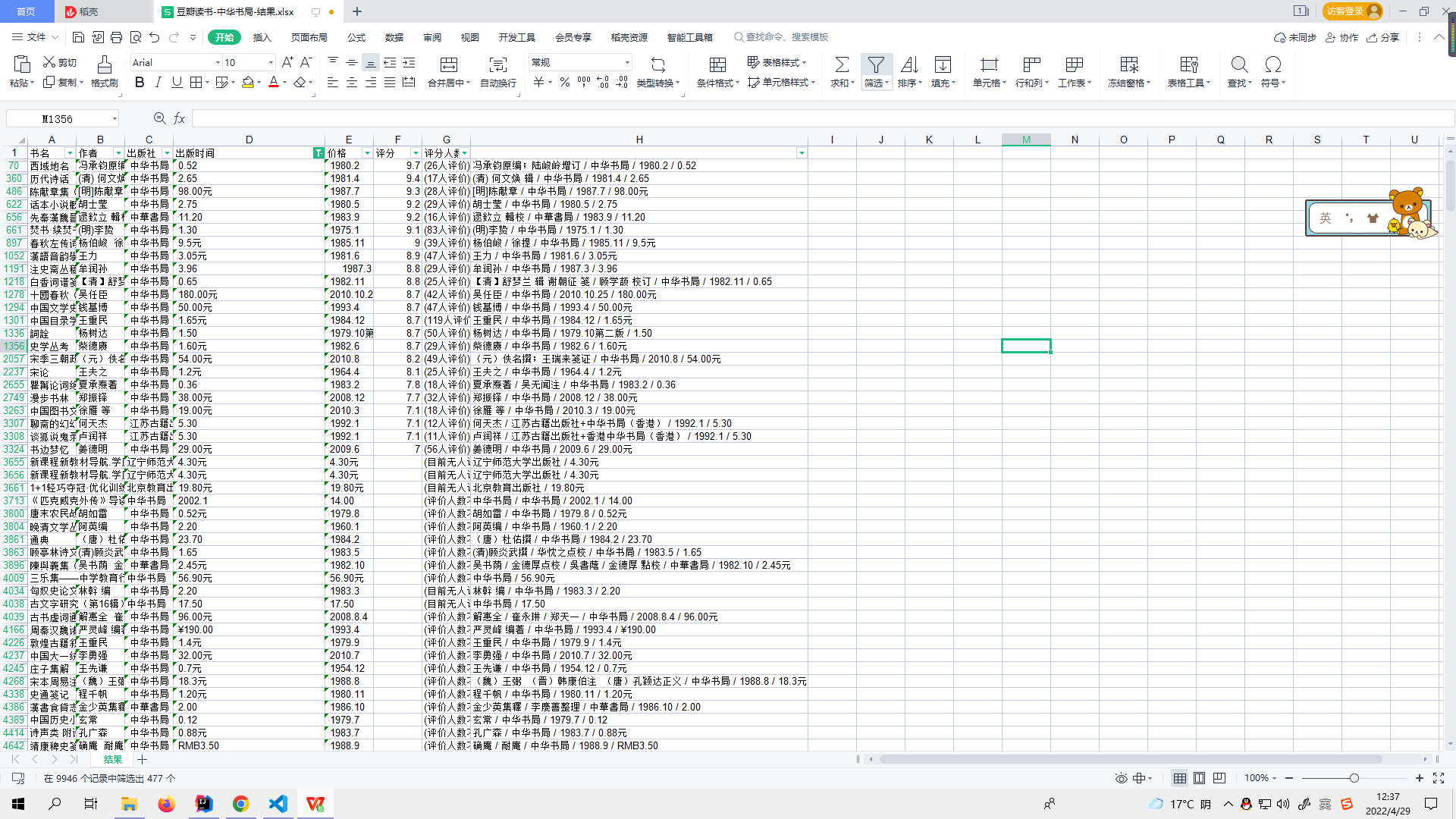Sinestro 2022-04-29 12:38 采纳率: 87.5%浏览 211

# 请问Python正则表达式如何匹配连续的四位数字

###### 问题相关代码，请勿粘贴截图
``````elif dataIndex == 0:
i.find('.')
i.find('元')
i.find('HKD')
i.find('-')
dict_Two["出版社"] = dataStringOne
if i.find('.') != -1:
dict_Two["价格"] = dataStringTwo
elif i.find('元') != -1:
dict_Two["价格"] = dataStringTwo
elif i.find('HKD') != -1:
dict_Two["价格"] = dataStringTwo
elif i.find('-') != -1:
dict_Two["出版时间"] = dataStringTwo
else:
dict_Two["出版时间"] = dataStringTwo

``````
###### 运行结果及报错内容• 写回答

#### 悬赏问题

• ¥15 关于selenium网页闪退
• ¥15 怎么用PYTHON解类似于x = y+zx + cy = x+zy + v的方程
• ¥300 FLASH AS2.0制作一个类似手机上下滑动一样的效果
• ¥15 为什么使用openFoam 中的icoFoam计算圆柱扰流时出现浮点数例外（核心已转储）
• ¥15 51单片机外部中断控制数码管
• ¥15 创建网页里面的图片显示不出来
• ¥15 语音控制的编写？(语言-c#)
• ¥15 matlab输入书上的代码运行错误
• ¥15 matlab怎么做三维曲面
• ¥50 sph光滑粒子法能否解决阶跃性的问题